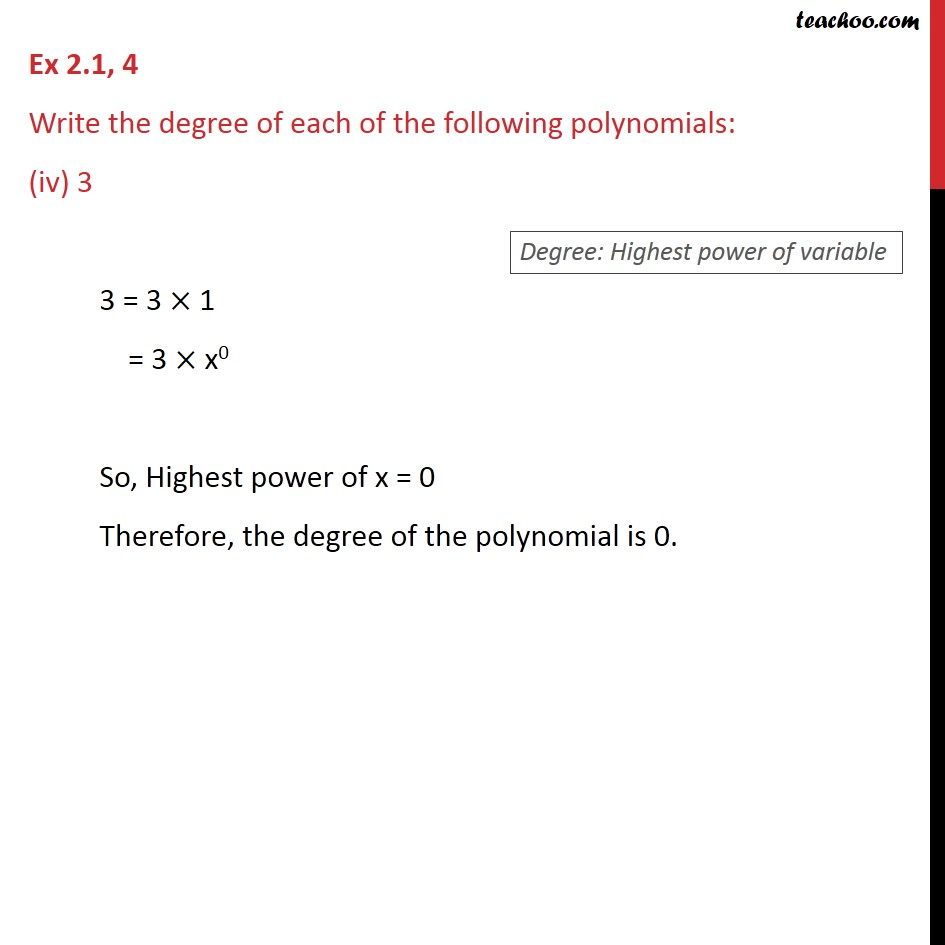1. Chapter 2 Class 9 Polynomials (Term 2)
2. Serial order wise
3. Ex 2.1

Transcript

Ex 2.1, 4 Write the degree of each of the following polynomials: (iv) 3 3 = 3 1 = 3 x0 So, Highest power of x = 0 Therefore, the degree of the polynomial is 0.